Feeds:
Posts

# Formal grammar

For the rules of a formal language as used in logic see Formation rules.

In theoretical computer science, a formal grammar (sometimes simply called a grammar) is a set of formation rules that describe which strings formed from the alphabet of a formal language are syntactically valid within the language. A grammar only addresses the location and manipulation of the strings of the language. It does not describe anything else about a language, such as its semantics (i.e. what the strings mean).

One of the fields of theoretical computer science that is concerned with the properties of formal grammars and languages and the relationships between them is called formal language theory. Its applications are found in computer science, linguistics, formal semantics, and other areas.

A grammar consists of a set of string rewriting rules with an assigned start symbol; the language described is the set of strings that can be generated by applying these rules arbitrarily, starting with the start symbol.

Therefore, a grammar is usually thought of as a language generator; but it can also be used as the basis for a recognizer that determines for any given string whether it is grammatical (i.e. belongs to the language). Whether this can effectively be done depends on the type of grammar used. To describe such recognizers, formal language theory uses separate formalisms, known as automata.

The process of recognizing a string by constructing a combination of applications of rules that generate it is known as parsing. Most languages have very compositional semantics, i.e. the meaning of their utterances is structured according to their syntax; therefore, the first step to describing the meaning of an utterance in language is to analyze it and look at its analyzed form (known as its parse tree in computer science, and as its deep structure in generative grammar).

##  Introductory example

A grammar mainly consists of a set of rules for transforming strings. (If it only consisted of these rules, it would be a semi-Thue system.) To generate a string in the language, one begins with a string consisting of only a single start symbol, and then successively applies the rules (any number of times, in any order) to rewrite this string. The language consists of all the strings that can be generated in this manner. Any particular sequence of legal choices taken during this rewriting process yields one particular string in the language. If there are multiple ways of generating the same single string, then the grammar is said to be ambiguous.

For example, assume the alphabet consists of a and b, the start symbol is S and we have the following rules:

1.2.then we start with S, and can choose a rule to apply to it. If we choose rule 1, we obtain the string aSb. If we choose rule 1 again, we replace S with aSb and obtain the string aaSbb. This process can be repeated at will until all occurrences of S are removed, and only symbols from the alphabet remain (i.e., a and b). For example, if we now choose rule 2, we replace S with ba and obtain the string aababb, and are done. We can write this series of choices more briefly, using symbols:. The language of the grammar is the set of all the strings that can be generated using this process:.

##  Formal definition

###  The syntax of grammars

In the classic formalization of generative grammars first proposed by Noam Chomsky in the 1950s, a grammar G consists of the following components:

• A finite set N of nonterminal symbols.
• A finite set Σ of terminal symbols that is disjoint from N.
• A finite set P of production rules, each rule of the formwhere * is the Kleene star operator anddenotes set union. That is, each production rule maps from one string of symbols to another, where the first string contains at least one nonterminal symbol. In the case that the second string is the empty string – that is, that it contains no symbols at all – in order to avoid confusion, the empty string is often denoted with a special notation (often λ, e or ε).
• A distinguished symbol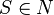that is the start symbol.

A grammar is formally defined as the ordered quad-tuple (N,Σ,P,S). Such a formal grammar is often called a rewriting system or a phrase structure grammar in the literature.

###  The semantics of grammars

The operation of a grammar can be defined in terms of relations on strings:

• Given a grammar G = (N,Σ,P,S), the binary relation(pronounced as “G derives in one step”) on strings inis defined by:• the relation(pronounced as G derives in zero or more steps) is defined as the transitive closure of• the language of G, denoted as, is defined as all those strings over Σ that can be generated by starting with the start symbol S and then applying the production rules in P until no more nonterminal symbols are present; that is, the set.

Note that the grammar G = (N,Σ,P,S) is effectively the semi-Thue system, rewriting strings in exactly the same way; the only difference is in that we distinguish specific nonterminal symbols which must be rewritten in rewrite rules, and are only interested in rewritings from the designated start symbol S to strings without nonterminal symbols.

###  Example

For these examples, formal languages are specified using set-builder notation.

Consider the grammar G where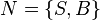,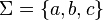, S is the start symbol, and P consists of the following production rules:

1.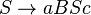2.3.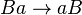4.Some examples of the derivation of strings inare:

•••(Note on notation:reads “L generates R by means of production i” and the generated part is each time indicated in bold.)

This grammar defines the languagewhere an denotes a string of n consecutive a‘s. Thus, the language is the set of strings that consist of 1 or more a‘s, followed by the same number of b‘s, followed by the same number of c‘s.

##  The Chomsky hierarchy

Main article: Chomsky hierarchy

When Noam Chomsky first formalized generative grammars in 1956, he classified them into types now known as the Chomsky hierarchy. The difference between these types is that they have increasingly strict production rules and can express fewer formal languages. Two important types are context-free grammars (Type 2) and regular grammars (Type 3). The languages that can be described with such a grammar are called context-free languages and regular languages, respectively. Although much less powerful than unrestricted grammars (Type 0), which can in fact express any language that can be accepted by a Turing machine, these two restricted types of grammars are most often used because parsers for them can be efficiently implemented. For example, all regular languages can be recognized by a finite state machine, and for useful subsets of context-free grammars there are well-known algorithms to generate efficient LL parsers and LR parsers to recognize the corresponding languages those grammars generate.

###  Context-free grammars

A context-free grammar is a grammar in which the left-hand side of each production rule consists of only a single nonterminal symbol. This restriction is non-trivial; not all languages can be generated by context-free grammars. Those that can are called context-free languages.

The language defined above is not a context-free language, and this can be strictly proven using the pumping lemma for context-free languages, but for example the language(at least 1 a followed by the same number of b‘s) is context-free, as it can be defined by the grammar G2 with,, S the start symbol, and the following production rules:

1.2.A context-free language can be recognized in O(n3) time (see Big O notation) by an algorithm such as Earley’s algorithm. That is, for every context-free language, a machine can be built that takes a string as input and determines in O(n3) time whether the string is a member of the language, where n is the length of the string. Further, some important subsets of the context-free languages can be recognized in linear time using other algorithms.

###  Regular grammars

In regular grammars, the left hand side is again only a single nonterminal symbol, but now the right-hand side is also restricted. The right side may be the empty string, or a single terminal symbol, or a single terminal symbol followed by a nonterminal symbol, but nothing else. (Sometimes a broader definition is used: one can allow longer strings of terminals or single nonterminals without anything else, making languages easier to denote while still defining the same class of languages.)

The language defined above is not regular, but the language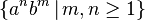(at least 1 a followed by at least 1 b, where the numbers may be different) is, as it can be defined by the grammar G3 with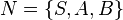,, S the start symbol, and the following production rules:

1.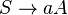2.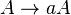3.4.5.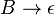All languages generated by a regular grammar can be recognized in linear time by a finite state machine. Although, in practice, regular grammars are commonly expressed using regular expressions, some forms of regular expression used in practice do not strictly generate the regular languages and do not show linear recognitional performance due to those deviations.

###  Other forms of generative grammars

Many extensions and variations on Chomsky’s original hierarchy of formal grammars have been developed, both by linguists and by computer scientists, usually either in order to increase their expressive power or in order to make them easier to analyze or parse. Some forms of grammars developed include:

• Tree-adjoining grammars increase the expressiveness of conventional generative grammars by allowing rewrite rules to operate on parse trees instead of just strings.
• Affix grammars and attribute grammars allow rewrite rules to be augmented with semantic attributes and operations, useful both for increasing grammar expressiveness and for constructing practical language translation tools.

##  Analytic grammars

Though there is a tremendous body of literature on parsing algorithms, most of these algorithms assume that the language to be parsed is initially described by means of a generative formal grammar, and that the goal is to transform this generative grammar into a working parser. Strictly speaking, a generative grammar does not in any way correspond to the algorithm used to parse a language, and various algorithms have different restrictions on the form of production rules that are considered well-formed.

An alternative approach is to formalize the language in terms of an analytic grammar in the first place, which more directly corresponds to the structure and semantics of a parser for the language. Examples of analytic grammar formalisms include the following: